# Force & Motion: Physics Lab

Instructor: Matthew Bergstresser
When learning about forces and motion, it is helpful to conduct a hands-on activity. In this lesson, we will do an activity relating force and motion by analyzing a mass that is swung in a horizontal circle.

## Force and Motion Lab

The following activity is designed to show the relation between force and motion by determining the value of a mass which is moving at constant speed in a circular path.

## Materials Required

• A thin plastic tube
• 1 meter of nearly massless string
• A rubber stopper of unknown mass
• An assortment of metal washers totaling 1200 g
• A mass scale
• A timer
• Red tape

## Procedure

1. Slide string through thin plastic tube and securely attach rubber mass to string at one end.

2. Put piece of red tape on string 0.5 m from rubber mass.

3. Slide plastic tube to the bottom of the red tape and put another piece of red tape on the string at the bottom of the plastic tube.

4. Attach 0.5 g of washers to other end of string.

5. Hold on to plastic tube and swing rubber mass around your head so that the pieces of red tape stay at the top and bottom of the red tube.6. When constant velocity has been reached (both pieces of red tape aren't moving), start the stopwatch and count 10 revolutions. Stop the stopwatch at 10 revolutions and slowly bring the rubber mass to a stop.

7. Weigh the washers.

8. Repeat steps 5-8 until you have 5 different masses of washers and a maximum washer mass of at least 1000 g.

## Data

The measurements we have to take are the mass of the washers for each new set of washers, and the time it took for 10 revolutions. The data for this is shown in Data Table 1.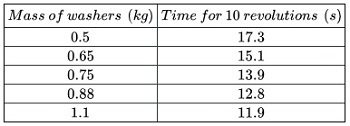## Theory

The hanging washers put tension on the string. This tension force is exerted through the entire string and acts as the centripetal force, which is the force acting towards the center of the circle, allowing the rubber stopper to move in a circular path. Even though the rubber stopper moves with constant speed in its circular path, it is accelerating towards the center of the circle. This is known as a centripetal acceleration. This acceleration demonstrates that there is an unbalanced force on the mass, and that force is the tension in the string.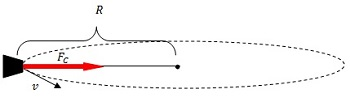Gravity is also acting on the mass, but it is in the vertical direction and not in the line of the centripetal force. Technically, the mass cannot be moving perfectly horizontally because there has to be a force to counter the gravitational force. The string is slightly angled to give a small vertical component of tension to counter the weight of the mass. We can ignore that here because there is no vertical acceleration.

The graph of the square of the speed (v2) vs centripetal force (weight of washers) gives us the ratio of the radius of the circle to the mass of the rubber stopper, which is the slope of the best-fit line through the data.

## Calculations and Graph

The speed equation is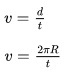• v = speed in m/s
• d is distance the rubber stopper moves in its path in 1 revolution in meters
• R is the radius of the circle in meters
• t is the time for 1 revolution in seconds (each time in the data table divided by 10 because of the 10 revolutions)

The weight of the washers is the mass of the washers times the acceleration due to gravity (9.8 m/s2).

This information is tabulated in Data Table 2.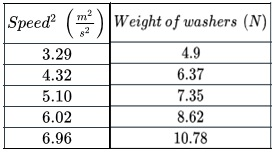We will graph this data, and draw a best-fit line through it.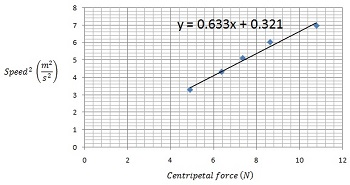## Analysis

We can see from the graph that the data plotted is linear. In fact, the slope of the line is given in the equation of the line, which is 0.633 m2/s2 / N. Let's analyze what these units reduce to.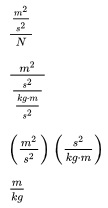The equation for centripetal force is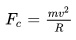which can be rearranged to solve for v2/Fc.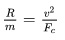To unlock this lesson you must be a Study.com Member.

### Register to view this lesson

Are you a student or a teacher?

### Unlock Your Education

#### See for yourself why 30 million people use Study.com

##### Become a Study.com member and start learning now.
Back
What teachers are saying about Study.com

### Earning College Credit

Did you know… We have over 160 college courses that prepare you to earn credit by exam that is accepted by over 1,500 colleges and universities. You can test out of the first two years of college and save thousands off your degree. Anyone can earn credit-by-exam regardless of age or education level.